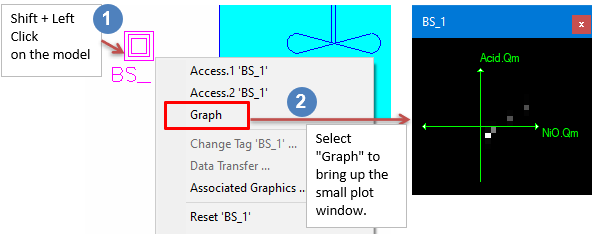# Bivariate Statistics

Navigation: Models ➔ Statistical Models ➔ Bivariate Statistics

## General Description

This model is used in Dynamic Simulation only.

The Bivariate Statistics model can be used to calculate and display statistical information about two variables and their correlation.

1. The bivariate statistics graphic can be displayed by holding down the shift key and left mouse clicking on the Bivariate Statistics graphical symbol.
2. From the menu, select "Graph" and place on the flowsheet.## Data Sections

The default sections and variable names are described in detail in the following tables. The default Bivariate Statistics access window consists of 2 sections. This number may increase or decrease, based on user configuration.

### Summary of Data Sections

1. BivariateStats tab - Contains general information relating to the unit.
2. Info tab - Contains general settings for the unit and allows the user to include documentation about the unit and create Hyperlinks to external documents.

### Bivariate Statistics Page

Unit Type: BivariateStats - The first tab page in the access window will have this name.

 Tag (Long/Short) Input / Calc Description/Calculated Variables / Options Tag Display This name tag may be modified with the change tag option. Condition Display OK if no errors/warnings, otherwise lists errors/warnings. ConditionCount Display The current number of errors/warnings. If condition is OK, returns 0. GeneralDescription / GenDesc Display This is an automatically generated description for the unit. If the user has entered text in the 'EqpDesc' field on the Info tab (see below), this will be displayed here. If this field is blank, then SysCAD will display the unit class ID. Requirements On Tick box When ticked, the model will log data and perform calculations. StatTag1 Input The first tag which will be logged, Variable 1. This will be graphed on the x-axis and calculations performed on its values. StatTag2 Input The second tag which will be logged, Variable 2. This will graphed on the y-axis and calculations performed on its values. Reset Button This will reset the calculations and graph. Auto_Reset Tick box If ticked, the calculations and graph will be reset at the beginning of every model run. Graph_x_Minimum / GraphMinx Input The minimum value to be shown on the x-axis of the graph. Any values lower than this will not be shown on the graph. Graph_x_Maximum / GraphMaxx Input The maximum value to be shown on the x-axis of the graph. Any values higher than this will not be shown on the graph. Graph_x_Minimum / GraphMiny Input The minimum value to be shown on the y-axis of the graph. Any values lower than this will not be shown on the graph. Graph_y_Maximum / GraphMaxy Input The maximum value to be shown on the y-axis of the graph. Any values higher than this will not be shown on the graph. Correlation / Corr Calc The calculated correlation between the two tags StatTag1 and StatTag2. A value of 1 means they are perfectly correlated. Variable 1 Value1 / Val1 Calc The current value of Variable 1 (StatTag1). Average1 / Avg1 Calc The average of the logged values of Variable 1 since the model was last reset. StandardDeviation1 / StdDev1 Calc The standard deviation of the logged values of Variable 1 since the model was last reset. Minimum1 / Min1 Output The minimum value of Variable 1 since the model was last reset. Maximum1 / Max1 Output The maximum value of Variable 1 since the model was last reset. Variable 2 Value2 / Val2 Calc The current value of Variable 2 (StatTag2). Average2 / Avg2 Calc The average of the logged values of Variable 2 since the model was last reset. StandardDeviation2 / StdDev2 Calc The standard deviation of the logged values of Variable 2 since the model was last reset. Minimum2 / Min2 Output The minimum value of Variable 2 since the model was last reset. Maximum2 / Max2 Output The maximum value of Variable 2 since the model was last reset. Number_of_Records / N Output The number of pairs of values logged since the model was last reset.

## Adding this Model to a Project

Insert into Configuration file

Sort either by DLL or Group.

 DLL: ControlDyn.dll → Units/Links → Statistics: Bivariate Statistics or Group: General → Units/Links → Statistics: Bivariate Statistics